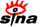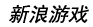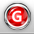下载新浪UT Game　客户端高速下载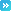推荐：全球新网游 | 网页游戏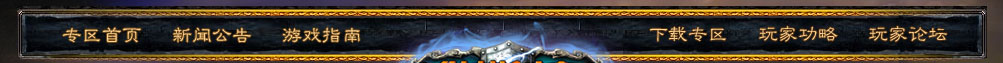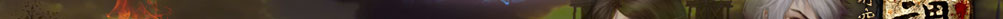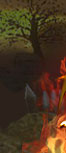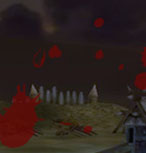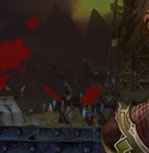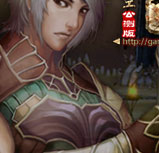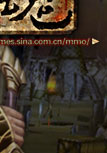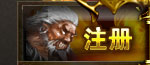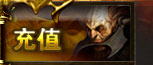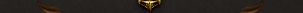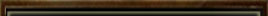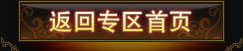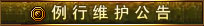为了保证游戏运行的稳定性及提升整体服务质量，《纵横时空》游戏全部服务器将每周二凌晨4：00-12：00进行例行停机维护， 停机期间给您带来的不便，敬请谅解。祝大家游戏愉快！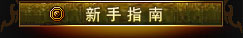登录游戏 创建角色 界面说明 基本操作 快 捷 键 角色属性 系统设置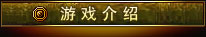背景故事 世界介绍 两大阵营 五大宗派 配置说明 下载安装 收费充值 Vista系统启动指南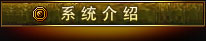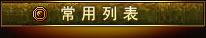游戏问题联系电话：            010-84799696 充值问题联系电话：客服电话：010-52926702/03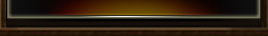# 天涯海角

 任务名称 接受等级 给予任务NPC 阵营需求 任务类型 海岛印象 >=26 门擎 新洛 主线任务 老渔民的麻烦 >=26 门擎 新洛 主线任务 牧渔者的恶行I >=26 老渔民阿旺 新洛 主线任务 牧渔者的恶行II >=26 老渔民阿旺 新洛 主线任务 门擎的忠告I >=26 门擎 新洛 主线任务 石人异变I >=26 门擎 新洛 主线任务 石人异变II >=26 海别 新洛 主线任务 汇报 >=26 海别 新洛 主线任务 海女王的踪迹 >=26 门擎 新洛 主线任务 门擎的忠告II >=26 门擎 新洛 主线任务 海蚌族神像 >=26 落魄的海女王 新洛 主线任务 门擎的忠告III >=26 门擎 新洛 主线任务 结盟 >=26 落魄的海女王 新洛 主线任务 走向沙平野 >=26 门擎 新洛 主线任务 曾经 >=26 钟正 正仪 主线任务 雷降的烦恼 >=26 钟正 正仪 主线任务 蚌之一族I >=26 雷降 正仪 主线任务 蚌之一族II >=26 雷降 正仪 主线任务 蚌之一族III >=26 钟正 正仪 主线任务 海蚌氏族的威胁IV >=26 钟正 正仪 主线任务 牧渔者的罪行I >=26 钟正 正仪 主线任务 牧渔者的罪行II >=26 霸桨 正仪 主线任务 牧渔者的罪行III >=26 高老伯 正仪 主线任务 牧渔者的罪行IV >=26 高老伯 正仪 主线任务 牧渔者的罪行V >=26 高老伯 正仪 主线任务 石人异变 >=26 霸桨 正仪 主线任务 祝由女神像 >=26 海别 正仪 主线任务 继续调查 >=26 钟正 正仪 主线任务 事情真相 >=26 海别 正仪 主线任务 建议 >=26 钟正 正仪 主线任务 迈入沙平野 >=26 钟正 正仪 主线任务 牧渔暴徒 >=26 老渔民阿旺 新洛 支线任务 防患于未然 >=26 海别 新洛 支线任务 祝由石板 >=26 海别 新洛 支线任务 恶徒 >=26 霸桨 新洛 支线任务 信使 >=26 门擎 新洛 支线任务 遗失的信件 >=26 信使 新洛 支线任务 相依为命I >=26 年轻人 新洛 支线任务 相依为命II >=26 小姑娘 新洛 支线任务 养颜佳品 >=27 英嫂 新洛 支线任务 为孙子报仇 >=26 高老伯 新洛 支线任务 寻找海里的宝藏 >=26 老渔民阿旺 新洛 支线任务 陈年老酒 >=26 高老伯 新洛 支线任务 谁不爱美 >=26 小姑娘 新洛 支线任务 夺回粮食 >=26 老渔民阿旺 新洛 支线任务 击杀首领 >=26 门擎 新洛 支线任务 海之一族的悲剧 >=26 落魄的海女王 新洛 支线任务 反击 >=26 落魄的海女王 新洛 支线任务 下酒菜 >=26 艾小财 正仪 支线任务 村民的委托 >=26 曲无畏 正仪 支线任务 探望甜草小妹 >=26 曲无畏 正仪 支线任务 甜草小妹的心事 >=26 甜草小妹 正仪 支线任务 夜明珠 >=26 远海花 正仪 支线任务 危机四伏 >=26 雷降 正仪 支线任务 除怪行动 >=26 霸桨 正仪 支线任务 采集珍珠 >=26 甜草小妹 正仪 支线任务 夺回神像 >=26 霸桨 正仪 支线任务 艺术爱好者 >=26 铁蛮 正仪 支线任务 可恶的石人 >=26 落魄的海女王 正仪 支线任务 鱼米之乡 >=26 曲无畏 正仪 支线任务 收集材料 >=26 艾小财 正仪 支线任务 消灭海蚌 >=26 钟正 正仪 支线任务 厉害的牧渔者 >=26 高老伯 正仪 支线任务 灾祸 >=26 高老伯 支线任务 异变危机I >=26 高老伯 支线任务 异变危机II >=26 高老伯 支线任务 异变危机III >=26 年轻人 支线任务 失落的财宝I >=26 支线任务 失落的财宝II >=26 霸桨 支线任务 失落的财宝III >=26 支线任务 祝由叛逆 >=30 信使 新洛 支线任务 奔赴天涯海角 >=26 古战阳 新洛 阵营任务 海别的求助 >=26 门擎 新洛 阵营任务 保护遗迹 >=26 海别 新洛 阵营任务 搬运罐子 >=26 海别 新洛 阵营任务 石人的形成 >=26 海别 新洛 阵营任务 汇报战果 >=26 海别 新洛 阵营任务 石碑的秘密 >=26 门擎 新洛 阵营任务 神奇的仪式 >=26 古老的石碑 新洛 阵营任务 奔赴天涯海角 >=26 洛青剑 正仪 阵营任务 寻求帮助 >=26 钟正 正仪 阵营任务 消灭石人 >=26 海别 正仪 阵营任务 修补小船 >=26 海别 正仪 阵营任务 石人的进化 >=26 海别 正仪 阵营任务 总结战况 >=26 海别 正仪 阵营任务 神秘的石碑 >=26 钟正 正仪 阵营任务 古老的仪式 >=26 古老的石碑 正仪 阵营任务 拯救者I >=27 支线任务 拯救者II >=26 兰姬碎沙 支线任务 拯救者III >=26 兰姬碎沙 支线任务 阿笨哥的计划I >=26 年轻人 支线任务 阿笨哥的计划II >=26 阿笨哥 支线任务 阿笨哥的计划III >=26 阿笨哥 支线任务 观日顶神殿I >=28 海别 支线任务 观日顶神殿II >=26 卓小燕 支线任务 观日顶神殿III >=26 卓小燕 支线任务 海女王自述 >=26 落魄的海女王 支线任务 探查底细 >=26 落魄的海女王 支线任务 寻找海蚌女王 >=26 落魄的海女王 支线任务 海蚌女王的下落 >=26 兰姬碎沙 支线任务 刺杀 >=26 落魄的海女王 支线任务 长久的战斗 >=26 落魄的海女王 支线任务 特殊信件 >=25 门擎 新洛 每日任务 特殊信件 >=25 钟正 正仪 每日任务 收集恐鸟爪 >=30 落魄的海女王 每日任务 美食 >=30 海别 每日任务 横岛争端 >=30 门擎 新洛 每日任务 横岛争端 >=30 钟正 正仪 每日任务 突击小队 >=27 钟正 正仪 每日任务 突击小队 >=27 门擎 新洛 每日任务 护送车队 >=26 高峻 新洛 每日任务 护送车队 >=26 潘明 正仪 每日任务 标记 >=27+<=29 海别 新洛 每日任务 军情汇报 >=28+<=30 门擎 新洛 每日任务 助人为乐 >=28+<=31 英嫂 新洛 每日任务 走访远亲 >=29+<=32 高老伯 新洛 每日任务 代理信使 >=30+<=32 信使 新洛 每日任务 标记 >=27+<=29 海别 正仪 每日任务 军情汇报 >=28+<=30 钟正 正仪 每日任务 助人为乐 >=28+<=31 艾小财 正仪 每日任务 走访远亲 >=29+<=32 高老伯 正仪 每日任务 代理信使 >=30+<=32 信使 正仪 每日任务 代理信使 >=33+<=35 信使 每日任务 稀有材料 >=26 英嫂 新洛 每日任务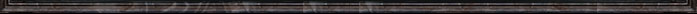留言 更多>>
 本新闻留言共条 显示 条
 登录名： 　密码： 　 匿名发表